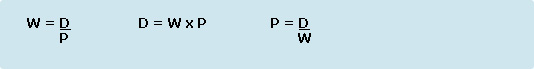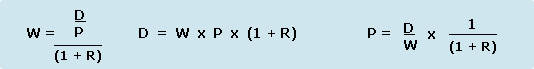# Wet and Dry Film Thickness Formulae

FORMULAS, CONVERSIONS AND EQUATIONS FOR
WET FILM THICKNESS, DRY FILM THICKNESS
& COVERAGE OF COATINGS

Easily convert and calculate the wet and dry film thickness and coverage of coatings applied to a substrate. Percentage of solids by volume in a coating will usually be known. Check the instructions for use of the coating or the 'Material Safety Data Sheet' (MSDS).

'Wet Film Thickness' (WFT) = W (This may be known, specified or calculated)
'Dry Film Thickness' (DFT) = D (This may be known, specified or calculated)
Percentage of Solids By Volume in Coating = P (This may be known, specified or calculated)
Percentage of Reducer By Volume added to Coating = R

Note that P & R should be expressed as a decimal less than 1. For example 72% would be 0.72

THICKNESS CALCULATIONS:

No Reducer or Thinner is added to Coating:If Reducer or Thinner is added to the coating:COVERAGE CALCULATIONS:

From the above equations, wet film thickness (WFT) may be easily calculated for a specified dry film thickness (DFT). If the wet film thickness is known the coverage of a coating in square feet per gallon may be calculated using the following formula:

Coverage in 'Square Feet Per Gallon' = 1604.17 Divided By the 'Wet Film Thickness in MILS

(** Above formula is based upon one gallon having a volume of 231 cubic inches)

For example: If the 'Wet Film Thickness is 10 MILS (0.010")

Coverage is: 1604.17 Divided by 10 which is 160.417 square feet per gallon.Manufacturing and Office Location:
GEI International, Inc.
100 Ball Street
East Syracuse, NY 13057 U.S.A.